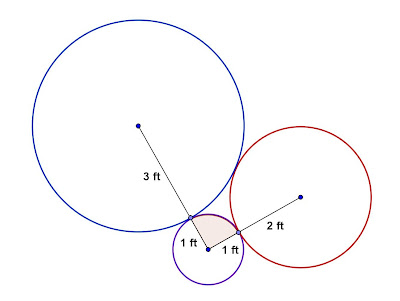## Monday, December 31, 2012

### Three Tangent Circles

Category: Plane Geometry

"Published in Suisun City, California, USA"

Three circles with radii 1, 2, and 3 ft. are externally tangent to one another, as shown in the figure. Find the area of the sector of the circle of radius 1 ft. that is cut off by the line segments joining the center of that circle to the centers of the other two circles.Photo by Math Principles in Everyday Life

Solution:

If you connect the centers of three circles by line segments, the figure is a triangle as shown belowPhoto by Math Principles in Everyday Life

The length of the sides of a triangle is calculated by the sum of the two radii that are tangent to each other. We notice that the sides of a triangle are the sides of a right triangle. Why? We can prove this by Pythagorean Theorem as follows

Therefore, a triangle is a right triangle. The right angle is located at the opposite side of hypotenuse which is 5 ft. The angle of the sector for the circle with a radius of 1 ft. is a right angle also or 90º.

Note: If a triangle is not a right triangle, then we have to use the Cosine Law in order to get the angle which is located at the opposite side of hypotenuse.

Since we know the angle of a sector of a circle with a radius of 1 ft., then we can calculate the area of the sector as follows

where: r = radius of a sector

θ = angle of a sector in radians (a unit less angle)

The value of θ in radians is calculated as follows

Therefore, the area of a sector is

## Sunday, December 30, 2012

### Exact Equation - Arbitrary Constant

Category: Differential Equations, Integral Calculus, Differential Calculus

"Published in Newark, California, USA"

Solve for the particular solution for

when x = 0, y = 2.

Solution:

The first that we have to do is to check the above equation if it is exact or not as follows

Let
then

Let
then

Since

then the given equation is Exact Equation. The solution for the above solution is F = C. Consider the given equation

Let
and

Integrate the partial derivative of the first equation above with respect to x, we have

Since we are integrating the partial derivatives, then another unknown function, T(y) must be added. If

then

To solve for T'(y), equate

To solve for T(y), integrate on both sides of the equation with respect to y, as follows

Since the arbitrary constant is already included in F = C, then we don't have to add the arbitrary constant in the above equation. Therefore,

The general solution of the equation is

To solve for the value of C, substitute x = 0 and y = 2 to the above equation, we have

Therefore, the particular solution of the equation is

## Saturday, December 29, 2012

### Indeterminate Form - One Raised Infinity

Category: Differential Calculus

"Published in Newark, California, USA"

Evaluate

Solution:

Consider the given equation

Substitute the value of x to the above equation

One raised to infinity is also another type of Indeterminate Form and it is not accepted as a final answer in Mathematics. We know that one raised to any number is always equal to one but in this case, one raised to infinity is not always equal to one, that's why 1 is also an Indeterminate Form. In this type of Indeterminate Form, we cannot use the L'Hopital's Rule because the L'Hopital's Rule is applicable for the Indeterminate Forms like 0/0 and ∞/∞. Since the above equation involves exponential function, we can rewrite the equation as follows

let

Take natural logarithm on both sides of the equation

Substitute the value of x to the above equation

Since the Indeterminate Form is ∞∙0, we have to rewrite the equation again as follows

Substitute the value of x to the above equation

Since the Indeterminate Form is 0/0, then we can now use the L'Hopital's Rule as follows

Substitute the value of x to the above equation

Take inverse natural logarithm on both sides of the equation

Therefore,